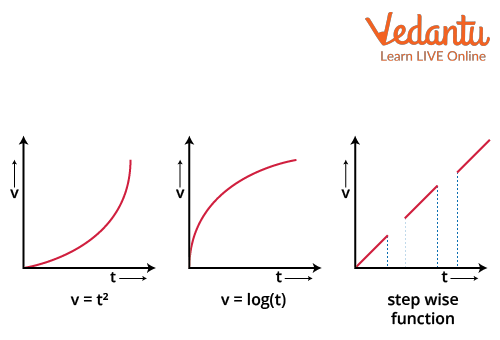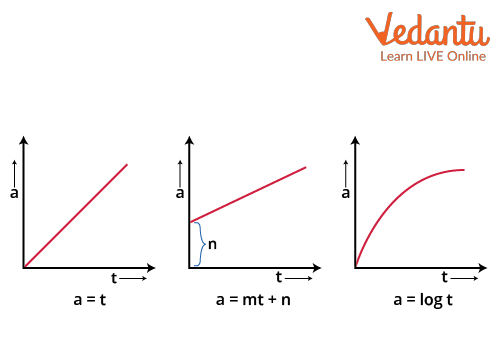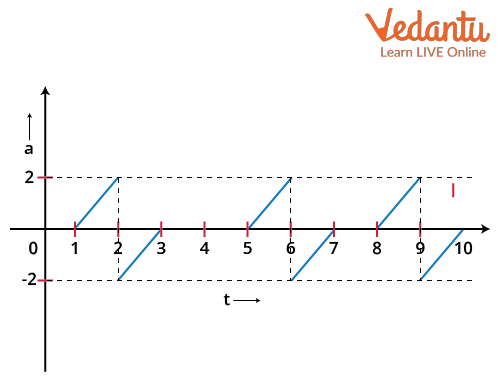Courses
Courses for Kids
Free study material
Free LIVE classes
More

# Non Uniformly Accelerated Motion for JEELIVE
Join Vedantu’s FREE Mastercalss

## Introduction to Non Uniformly Accelerated Motion

During a train journey, you may have noticed that when the train leaves the platform, a sudden jerk is experienced. Then with its speed increasing gradually, you can feel a force at your back constantly. When it starts slowing down due to a signal, you can feel the force pushing you forward. The train performs this kind of motion several times throughout its journey. This entire motion of the train is an example of non uniformly accelerated motion. This also happens when you are moving in car/bike heavy traffic. You can feel the back and forth motion all the time. We are going to discuss it here in detail.

## Non-Uniform Acceleration

We know what acceleration is. It is the rate of change of velocity, i.e., $a=\dfrac{d v}{d t}$. Now if the acceleration of an object in a particular motion is not constant, we call its acceleration a non-uniform acceleration. So, the “a-t” graph will always be non-linear and change with time.

Just consider the example we started with, the train’s motion. When the train was starting, it put in a lot of acceleration to get out of the static inertia and go on accelerating until the signal came; the train had to slow it down and again a lot of change in acceleration occurred. Therefore, in the entire trip, the acceleration of the train is not constant.

## Important Formulas and Derivation

In this section, we provide some non-uniform acceleration formulas that may be useful for solving related problems. If v is the velocity of a particle, and “a” be its acceleration (rate of change of velocity), then,

$a=\dfrac{d v}{d t}=\dfrac{d^{2} x}{d t^{2}}=\dfrac{d x}{d t} \dfrac{d v}{d x}=v \dfrac{d v}{d x}$

As velocity (rate of change of displacement),

$v=\dfrac{d x}{d t}$

This is the basic step of formulas to be used.

1. If the given acceleration is a function of time, then,

Given,

$a=\dfrac{d v}{d t}$

$\Rightarrow a d t=d v$

$\Rightarrow \int a d t=\int d v=v$

Now,

$v=\dfrac{d x}{d t}$

$\Rightarrow d x=v d t \Rightarrow \int d x=\int v d t$

$\Rightarrow x=\int v d t$

2. If the acceleration is a function of velocity,

Given,

$a=\dfrac{d v}{d t}$

$\Rightarrow \int v d v=\int a d x$

We can use these two cases to solve the non-uniform accelerated motion of a particle.

## Relevant Graphs of Non Uniformly Accelerated Motion

Here, we are going to discuss a few important graphs for this concept.

### 1. Velocity Time Graph for Non Uniformly Accelerated Motion

Note that in the v-t graph, the slope at any point ‘t’ is the acceleration at that point. As the slope of the graph is not constant, it cannot be linear.

Here are some possible v-t graphs.Velocity-Time Graph for some Non-Uniform Acceleration

For the three cases, observe that all the graphs are not linear. Hence, their slope would not be constant, which is non-uniform acceleration. We can physically calculate the acceleration and check its non-uniform accelerated motion.

### 2. Non Uniformly Accelerated Motion Graph (a-t)

As we know, acceleration is not constant. The graphs will not be lines parallel to the x-axis for the entire time. Now, we will take a few examples of the graphs.Acceleration-Time Graph for Non-Uniform Accelerated Motion

For these cases, $\dfrac{d a}{d t} \neq 0$. It is another way to check the non-uniformity in the acceleration of these motions. Now, here is an interesting example of a graph.

Note: Area under a-t graph represents velocity, while the area under v-t graph represents displacement.

## Solved Examples on Non Uniformly Accelerated Motion

1. Consider you are moving in a motorcycle in a heavy traffic jam. The figure below shows the “a-t” graph of your motion. Find out the highest velocity in the motion.Figure for the Question

Ans: In order to find the maximum velocity, we first need to find the relationship between the acceleration and the time. This can be found by writing the equation of the line between the y and x axis for the above graph.

We know that the equation of a line is given as

y=mx+c

Here, m is the slope and c is the y intercept. For our case, y will be a and x will be t. Now let’s consider the positive interval from t=1 to 2 sec. In this interval, the slope is 2. Also, since the line is starting from t=1, the equation will be a=2(t-1). We will be assuming that the initial velocity is 0 since it is not given.

Integrating both sides of the equation gives

\begin{align} &\int a d t=\int_{1}^{2} 2(t-1) d t \\ &\int d v=2\left[\dfrac{t^{2}}{2}-t\right]_{1}^{2} \\ &v=2\left[\dfrac{4}{2}-2-\dfrac{1}{2}+1\right] \\ &v=1~\dfrac{\mathrm{m} }{ \mathrm{s}} \end{align}

Since the other interval from t=2 to t=3 has a negative acceleration, the velocity will increase from t=1 to t=2 and will have a maximum value at t=2 which will be 1$\dfrac{\mathrm{m} }{ \mathrm{s}}$.

So, the maximum velocity is 1$\dfrac{\mathrm{m} }{ \mathrm{s}}$.

2. Given a non uniformly accelerated motion of a particle by $a(t)=-\dfrac{1}{t^{2}} m s^{-2}$, and after 1 second, the particle has velocity 1$\dfrac{m}{s}$ at origin, try to find out its position after 10 seconds.

Ans: Given acceleration of the particle is

$a(t)=-\dfrac{1}{t^{2}} m s^{-2}$

Now,

\begin{align} &v(t)=\int a(t) d t \\ &\Rightarrow v(t)=\int\left(-\dfrac{1}{t^{2}}\right) d t \\ &\Rightarrow v(t)=\dfrac{1}{t}+c_{1} \end{align}

where c1=constant

We have

$v(t=1)=2=\frac{1}{1}+c_{1} \Rightarrow c_{1}=1$

Now,

$\Rightarrow v(t)=\frac{1}{t}+1$

and

$x(t)=\int v(t) d t=\int\left(\frac{1}{t}+1\right) d t=\log (t)+t+c_{2}$

$c_{2}=\operatorname{cons} \tan t$

Given, $x(t=1)=0=\log (1)+1+c_{2} \Rightarrow c_{2}=-1$

So, $x(t)=\log (t)+t-1$

$x(10)=\log (10)+10-1 \approx 2.3+9=11.3 \mathrm{~m}$

The particle is at 11.3 m after 10 seconds.

## Conclusion

Motion takes place when there is a force acting on any particle and this force creates an acceleration of the body on which it is acting. Now, this acceleration could be uniform or non uniform. In uniform acceleration, the velocity of the body remains constant and in non-uniform acceleration, the velocity of any body changes with time. We refer to an object's acceleration as being non-uniform if it is not constant during a certain motion. As a result, the "a-t" graph will never be linear and will alter over time. Calculus can be used to solve problems relating to non-uniform acceleration.

Last updated date: 28th Sep 2023
Total views: 3.3k
Views today: 0.03k

## FAQs on Non Uniformly Accelerated Motion for JEE

1. A child throws a ball in the sky and it falls back after sometime. Is it non-uniform or uniform motion?

Irrespective of the direction of the ball, the gravitational pull or acceleration “g” will be always downwards with constant value. So, it is uniformly accelerated. If you consider “g” to be not constant as with increasing height, g decreases. So, in the course of the journey, g is not constant or non-uniform. However, if the maximum height of the ball is not too high, we can take it as constant. So, the answer quite depends on the scenario. If the initial velocity is too high, then it will be non-uniform.

2. Is the motion of the Pendulum non uniformly accelerated?

It also depends on the type of acceleration you are considering. A simple pendulum has net acceleration “g” but it has acceleration towards the trajectory of its motion and that depends on the position of the pendulum, so it changes with the position of the pendulum. There is another component along the string of the pendulum, outwards that also depends on the position. So, it depends on the question. If it says the net acceleration, then it is uniformly accelerated by “g”.

3. What is the importance of non-uniform acceleration in JEE?

This is a very score-friendly topic in the JEE exam. There will definitely be questions from this topic. There is no extra formula required for solving these kinds of questions, just the above-mentioned formula and good knowledge of Calculus are enough to solve. Moreover, this is a very common phenomenon of kinematics that happens everyday in your life and you can observe and calculate from your basic observations. We recommend you solve questions of kinematics on this particular topic to do it quickly in the exam.